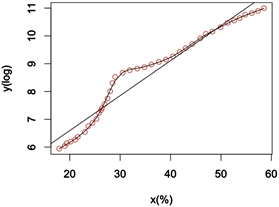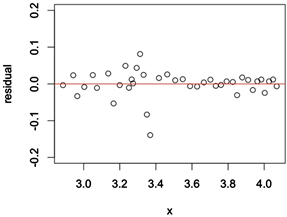#### 期刊菜单

Empirical Study on the Impact of Urbanization Rate on Economic Growth in China Based on Smooth Spline Es-timation
DOI: 10.12677/SA.2019.83065, PDF, HTML, XML, 下载: 659  浏览: 1,418  科研立项经费支持

Abstract: In the real world, the relationship between most variables is not linear. When using time series model to explore the relationship between urbanization rate and economic growth in China, the cointegration test fails, showing that there is no linear cointegration relationship between them. Therefore, this paper uses smooth spline estimation to study the relationship between urbanization rate and economic growth in China, and compares it with the classical linear regression method on the actual data set. Through empirical analysis, it is found that the fitting effect of smooth spline is better than that of linear regression model.

1. 引言

2. 自然三次样条模型

$f\left(t\right)=g\left(t\right)={d}_{i}{\left(t-{t}_{i}\right)}^{3}+{c}_{i}{\left(t-{t}_{i}\right)}^{2}+{b}_{i}\left(t-{t}_{i}\right)+{a}_{i}$

${t}_{i}\le t\le {t}_{i+1},i=0,1,\cdots ,n$ (1)

${y}_{i}=g\left({t}_{i}\right)+{\epsilon }_{i}$ (2)

$|i-j|\ge 2$。又令R维 $\left(n-2\right)×\left(n-2\right)$ 维对称矩阵，由元素 ${r}_{ij}$ 构成， $i=j=1,\cdots ,n-1$${r}_{i,i}=\frac{1}{3}\left({h}_{i+1}-{h}_{i}\right)$$i=1,\cdots ,n-1$${r}_{i,i+1}={r}_{i+1,i}=\frac{1}{6}{h}_{i}$$i=2,\cdots ,n-2$${r}_{i,j}=0$，若 $|i-j|\ge 2$。因此R是严格的正定矩阵，定义矩阵K： $K=Q{R}^{-1}{Q}^{\prime }$。当且仅当 ${Q}^{\text{T}}g=R\gamma$ 时， ${\int }_{a}^{b}{\left\{{g}^{″}\left(t\right)\right\}}^{2}\text{d}t={\gamma }^{\prime }R\gamma ={g}^{\prime }Kg$。满足上述条件时g和 $\gamma$ 可以完全确定一个自然三次样条函数。

$S\left(g\right)=\underset{i=1}{\overset{n}{\sum }}{\left({Y}_{i}-g\left({t}_{i}\right)\right)}^{2}+{\int }_{a}^{b}{\left\{{g}^{″}\left(x\right)\right\}}^{2}\text{d}x$ (3)

${\sum }^{\text{​}}{\left\{{Y}_{i}-g\left({t}_{i}\right)\right\}}^{2}={\left(Y-g\right)}^{\prime }\left(Y-g\right)$

$\begin{array}{c}S\left(g\right)={\left(Y-g\right)}^{\prime }\left(Y-g\right)+\lambda {g}^{\prime }Kg\\ ={g}^{\prime }\left(I+\lambda K\right)g-2{Y}^{\prime }g+{Y}^{\prime }Y\end{array}$

$S\left(g\right)$ 对g的偏导数，

$\frac{\partial S\left(g\right)}{\partial g}=2\left(I+\lambda k\right)g-2Y$

$\frac{\partial S\left(g\right)}{\partial g}=0$，可得 $g={\left(I+\lambda k\right)}^{-1}Y$$\gamma$ 的值可用 $\gamma ={R}^{-1}Kg$ 求得。在给定光滑参数 $\lambda$ 的值后，三次光滑样条可用以上向量形式表示。

$\text{GCV}\left(\alpha \right)={n}^{-1}\frac{{\sum }_{i=1}^{n}{\left\{{y}_{i}-\stackrel{^}{g}\left({t}_{i}\right)\right\}}^{2}}{{\left\{1-{n}^{-1}trA\left(\alpha \right)\right\}}^{2}}$

3. 实证分析

3.1. 协整检验

3.2. 光滑样条估计Figure 1. Fit of smooth Spline and linear regression

$\stackrel{^}{y}=\mathrm{exp}\left\{\stackrel{^}{g\left(x\right)}\right\}$Figure 2. Residual plot of smooth splineTable 2. Results of linear regression

$\stackrel{^}{y}=\text{exp}\left(4.075836+0.125853x\right)$

$MSE=\frac{1}{n}\underset{i=1}{\overset{n}{\sum }}{\left({y}_{i}-\stackrel{^}{g\left({t}_{i}\right)}\right)}^{2}$Table 3. Root MSE predicted by two types of methods

4. 主要结论

  Herderson, J.V. (2003) The Urbanization Process and Economic Growth: The So-What Question. Journal of Economical Growth, 8, 47-71.  沈坤荣, 蒋锐. 中国城市化对经济增长影响机制的实证研究[J]. 统计研究, 2007(6): 9-15.  闫晓红. 城市化水平与长期经济增长关系的实证分析——基于27个省份面板数据的考察[J]. 经济经纬, 2011(3): 25-28.  陈生长, 徐勇勇, 夏结来. 光滑样条非参数回归方法及医学应用[J]. 中国卫生统计, 1999(6): 342-345.